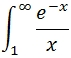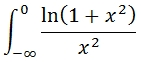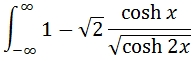# SQL Server 15-point Gauss-Kronrod quadrature

Updated: 31 March 2014

Use the scalar function QUAD to evaluate an infinite integral. QUAD can be used to evaluate integrals in the regions -Infinity to a, a to Infinity, or -Infinity to Infinity, where -Infinity < a < Infinity. QUAD uses 15-point Gauss-Kronrod quadrature.
Syntax
<@Func, nvarchar(max),>
,<@VarName, nvarchar(4000),>
,<@A, sql_variant,>
,<@B, sql_variant,>)
Arguments
@Func
the function to be integrated. @Func is a string containing any valid TSQL statement which includes a single variable that is the object of the integration. The variable name is defined in @VarName. @Func is of a type nvarchar or of any type which implicitly converts to nvarchar.
@VarName
the TSQL variable name. The variable name must start with '@'. @VarName must be of a type nvarchar of a type which implicitly converts to nvarchar.
@A
The lower limit of integration.
@B
The upper limit of integration.
Return Types
float
Remarks
·         If @A is not '-Inf' and @B is not 'Inf' then NULL will be returned.
·         If @A is '-Inf' then the function will be integrate from –8 to @B.
·         If @A is not '-Inf' then the function will be integrated from @A to 8.
·         @A can be any floating point number or '-Inf'.
·         @B can be any floating point number or 'Inf'.
·         If @Func contains an undeclared SQL variable and it is not defined in @VarName a NULL will be returned.
Example
In this example we want to evaluate the integral:SELECT
'SELECT EXP(-@x)/@x',   --@Func
'@x',                   --@VarName
1,                      --@A
'Inf'                   --@B
) as Integral

This produces the following result.
Integral
----------------------
0.21938393439552

In this example we want to evaluate the integral:SELECT
'SELECT LOG(1+POWER(@x,2))/POWER(@x,2)', --@Func
'@x',       --@VarName
'-Inf',     --@A
0           --@B
) as Integral

This produces the following result.
Integral
----------------------
3.14159265358684

In this example we want to evaluate the integral:Note that COSH, the hyperbolic cosine function, is not a built-in SQL Server function, but it is part of the XLeratorDB library.
SELECT
'SELECT 1 - SQRT(2)*wct.COSH(@x)/SQRT(wct.COSH(2*@x))', --@Func
'@x',    --@VarName
'-Inf', --@A
'Inf'    --@B
) as Integral

This produces the following result.
Integral
----------------------
-0.69314718056001

### SupportCopyright 2008-2021 Westclintech LLC         Privacy Policy        Terms of Service